# Brahmagupta-Fibonacci two-square identity

## Statement

### As an identity

For allin a commutative ring: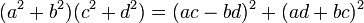### For products two at a time

In any commutative unital ring, ifandcan each be written as a sum of two squares, so can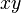.

More concretely, ifand, then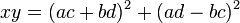.

### For products more than two at a time

In any commutative unital ring, ifare elements each of which can be written as a sum of two squares, so can the product.

Note that this follows by induction from the statement for products two at a time.

## Interpretations

### In terms of complex numbers

This identity is motivated by complex numbers. In fact, it is precisely the statement that the modulus-squared operation for complex numbers is multiplicative. To see this note that:

•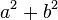is the modulus-squared of the complex number•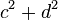is the modulus-squared of the complex number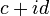.
•is the modulus-squared of the complex number.

### In terms of trigonometry

This is based on the interpretation in terms of complex numbers, and relates the formula to the angle sum formulas for sine and cosine. Fill this in later